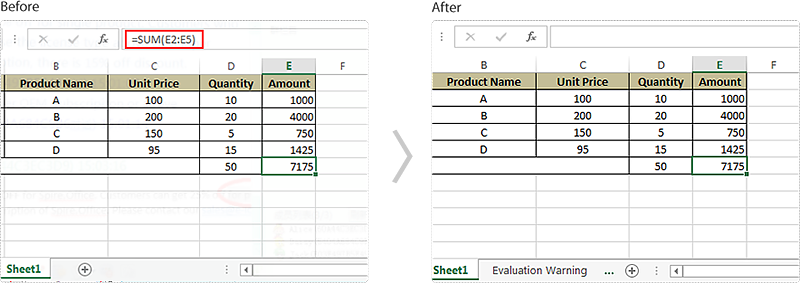# Hide Formulas When Protecting a Worksheet in C#

If your worksheet has some important formulas that you don’t want others to view, you may want to hide these formulas. This article demonstrates how to hide formulas when protecting a worksheet using Spire.XLS and C#.

The XlsRange.IsFormulaHidden property is used to determine if the formula will be hidden when the worksheet is protected. You can hide the formulas in a specific cell range by setting the XlsRange.IsFormulaHidden property to true, but note that the formulas can be hidden only if the worksheet is protected, they won’t be hidden if the workbook is protected while the worksheet is not.

```using Spire.Xls;
namespace HideFormulas
{
class Program
{

static void Main(string[] args)
{
//Initialize an object of Workbook class
Workbook workbook = new Workbook();

//Get the first worksheet
Worksheet sheet = workbook.Worksheets;

//Hide the formulas in the used range
sheet.AllocatedRange.IsFormulaHidden = true;

sheet.Protect("123");

//Save the file
workbook.SaveToFile("HideFormulas.xlsx", ExcelVersion.Version2013);

}
}
}
```

Screenshot: